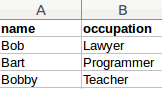# Pandas Data Structures

Python hosting: Host, run, and code Python in the cloud!

Pandas, a data analysis library, supports two data structures:

• Series: one-dimensional labeled arrays pd.Series(data)
• DataFrames: two-dimensional data structure with columns, much like a table.

Related course:
Data Analysis with Python and Pandas: Go from zero to hero

## Series

A series can be seen as a one-dimensional array. The data structure can hold any data type, that is includings strings, integers, floats and Python objects.

A very basic example is shown below, where it holds characters:

It can contain an integer list:

A pandas series can also contain a dictionary:

To get a single value use:

To get a subset:

You can also use operators on the series:

## Data Frames

The data frame datastructure is similar to a table. Data Frames are the most commonly used Pandas data structures. So how is it made?

Lets say you have the following table:Example Dataframe

Then you can represent that as a Python dictionary like this:

After that, you can create a new DataFrame object.

Unlike a dictionary, a Data Frame allows you to do all kinds of operations on the data quickly.
The complete code then becomes:

Data may be defined manually or loaded from:

Related course:
Data Analysis with Python and Pandas: Go from zero to hero

## Indexing / selection

You can do selection on a dataframe, first define a DataFrame.

You can select a column by it’s column header name

You can select a row by integer location

If you want to slice rows from the data frame, you can do that too:

## Data arithmetic

You can do many operations on Pandas data frames and series.
For example, you can apply scalars to a series.

Scalars can be applied to data frames too.

In the example below we create a dataframe with random numbers (using import numpy as np).
Then we apply a scalar to the data frame.